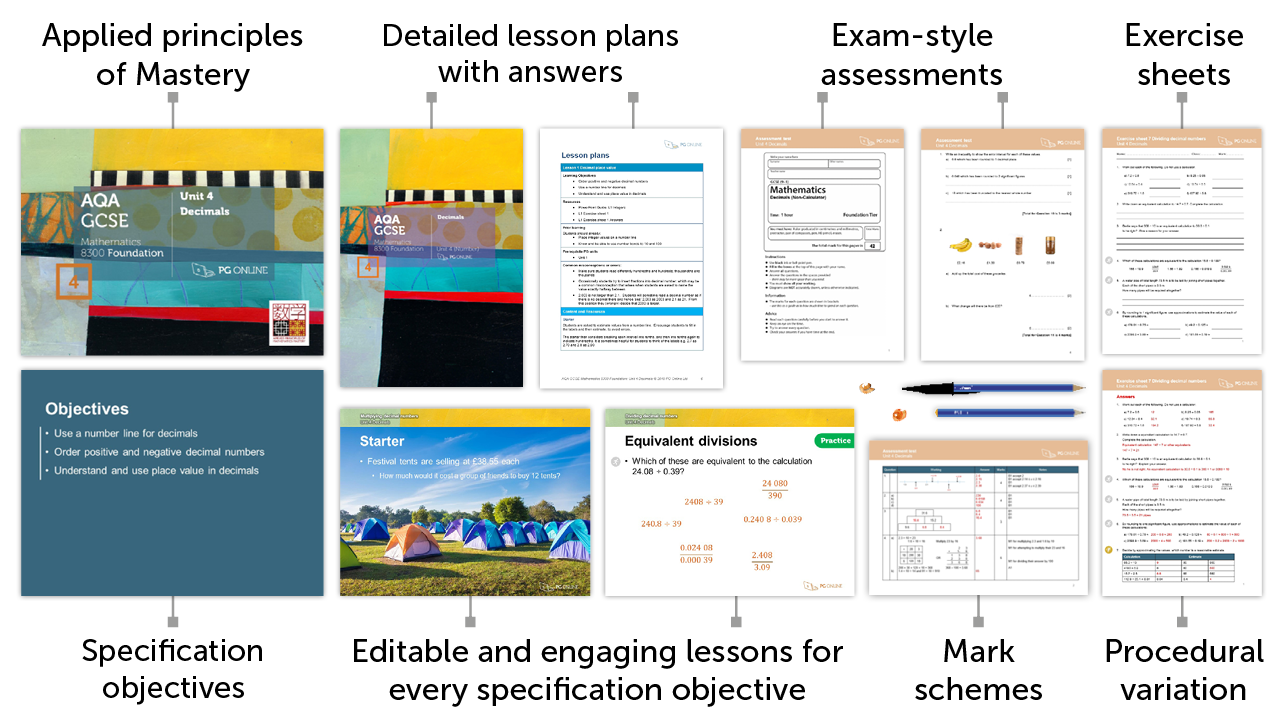# Mastery for GCSE AQA

### £75.00 + VAT+

10

## Inside the Unit...### Areas Covered

• Lesson 1: Ratio notation
• Lesson 2: Divide in a given ratio
• Lesson 3: Ratio and proportion
• Lesson 4: Solving problems with ratio
• Lesson 5: Ratio as a linear function
• Lesson 6: Map scales
• Unit assessment

There are 6 exercise sheets and an examination-style assessment test, each with answers included in this unit.

## Mathematics Mastery

Our resources apply the principles of Mastery throughout, creating a rich and deep learning experience. Find out more about Mastery with our quick guide.## Summary

With a strong emphasis on using techniques from bar modelling to develop mastery, this unit focuses on developing skills with ratio. Starting from the basics, the concept of ratio, its notation and the connection to fractions is developed. Simplifying ratio, dividing in a given ratio and solving ratio problems in context are explored and procedural variation is used to support this learning. Direct proportion is linked to ratio and students recognise, represent and use direct proportion to solve graded questions. Ratio as a linear function is presented graphically, and students interpret the gradient and use reasoning to solve questions in context. The unit closes with a lesson on scale factors and maps where the fluency of the earlier ratio work supports this application of ratio in a real-life context.

## What's included in the toolkit?The GCSE units have been written to satisfy the specification for the AQA 8300 Maths GCSE. Each unit contains:

• PowerPoint slides for each component
• Detailed lesson plans
• Learning objectives and outcomes
• Worksheets and homework activities with answers
• End-of-unit, exam-style assessment test with answers
• Other material and links to online resources

## What people say...

The lessons are designed to develop deep understanding so students master the mathematical concepts and techniques involved.

Heather Davis. Professional Development Lead, NCETM

As I am non-specialist teaching the new specification of AQA GCSE Chemistry for the first time this year, these resources have been invaluable to me. The presentations are thorough and well illustrated. The teacher guide and power point presentation leads you through the topics logically.

Helen Webb. Science teacher, Lutterworth College

## Inside the Unit...

### Areas Covered

• Lesson 1: Ratio notation
• Lesson 2: Divide in a given ratio
• Lesson 3: Ratio and proportion
• Lesson 4: Solving problems with ratio
• Lesson 5: Ratio as a linear function
• Lesson 6: Map scales
• Unit assessment

There are 6 exercise sheets and an examination-style assessment test, each with answers included in this unit.

## Mathematics Mastery

Our resources apply the principles of Mastery throughout, creating a rich and deep learning experience. Find out more about Mastery with our quick guide.• ### How to order

1. Add individual units to a draft order or download a blank order form below to complete manually

2. Using a draft order you can either:

2. Email us your complete order
3. Create a PDF
(to fax or email at a later date)

• ### Unit Pricing and Discounts

Each unit is individually priced for LIFE. Please view each unit for detail.

Discounts are cumulative based on the total of units licenced to your department.

All prices subject to further discounts and VAT.

There are no annual renewal fees.

 2-5 units 10% discount 6-11 units 15% discount 12-23 units 20% discount 24+ units 25% discount